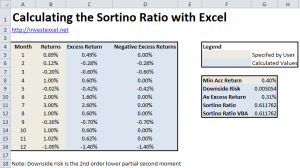# Calculate the Sortino Ratio with Excel

This Excel spreadsheet calculates the Sortino Ratio for an investment, a measure of risk-adjusted return. Investments that emphasize their Sortino Ratio often try to minimize their losses as a part of their trading strategy.

Investors can use a range of measures to gauge the suitability of investments.  The Sharpe Ratio, for example, measures the risk-efficiency of investments by using standard deviation to represent risk.  A disadvantage of this approach is that this penalizes both downside and upside volatility.  Given that investors don’t mind (and indeed welcome) large upwards movements in price, the Sharpe Ratio may not best represent investor psychology.  The Sortino Ratio, however, only penalizes downside risk, and is defined asThe Target Return is either a minimum acceptable return (as in the original definition), or a risk-free rate.  Lower Sortino Ratios signify investments with a greater risk of large losses and should be avoided by risk-averse investors.The Sortino Ratio was developed as a commercial measure by the investment industry, and does not have the academic heritage and strict mathematical definition of the Sharpe Ratio.  As such, several methods are commonly used to measure downside risk, including the semi standard deviation, or the square root of the 2nd lower partial moment.  The Excel spreadsheet uses the latter  (as suggested by Wikipedia) to represent downside risk.

There’s some uncertainty about whether you simply set all values above the minimum acceptable return to zero, or discard those values entirely when computing the downside risk.  Setting values above the MAR to zero reduces the computed risk; some feel that this is not consistent with the spirit of the Sortino Ratio and remove these values entirely instead.  Given the flexible definition of the Sortino Ratio, then as long as you use a consistent definition to compare the the risk efficiency of investments this may not matter.

## Sortino Ratio in VBA

```Function SortinoRatio(returns As Range, MAR As Variant) As Variant   Dim n As Integer Dim i As Integer Dim avgReturn As Double Dim mm2d As Double Dim downDev As Double   n = returns.Rows.Count avgReturn = WorksheetFunction.Average(returns) moment2d = 0 For i = 1 To n If returns(i) - MAR < 0 Then mm2d = mm2d + ((returns(i) - MAR) ^ 2) End If Next downDev = Sqr(mm2d / n) If downDev > 0 Then SortinoRatio = (avgReturn - MAR) / downDev Else SortinoRatio = "undefined" End If   End Function```

This Excel spreadsheet implements both a manual worksheet method and a VBA function to calculate the Sortino Ratio### 5 thoughts on “Calculate the Sortino Ratio with Excel”

1. Nice spreadsheet and VBA code. So how would you apply this to a portfolio of ETFs and decide how to allocate capital between them?

2. Excellent summary, well done.

3. I believe this is incorrect. The portfolio return is 8.78% yet this formula is using 0.71%, which is the average. Is that correct? Also, the downside deviation is .005054 but I am coming up with .004371, which is the STDEV(Negative Excess Column).

Am I off base, or is the poster incorrect?